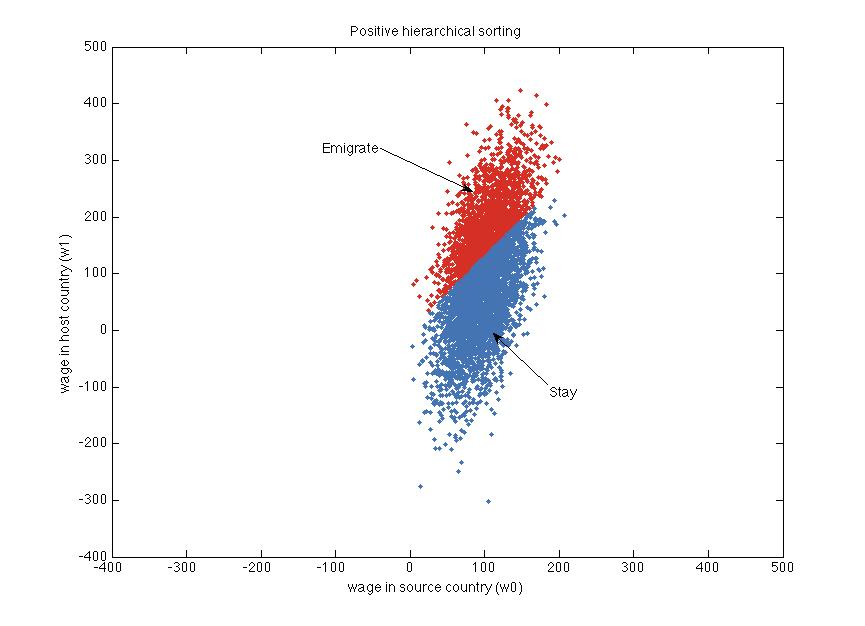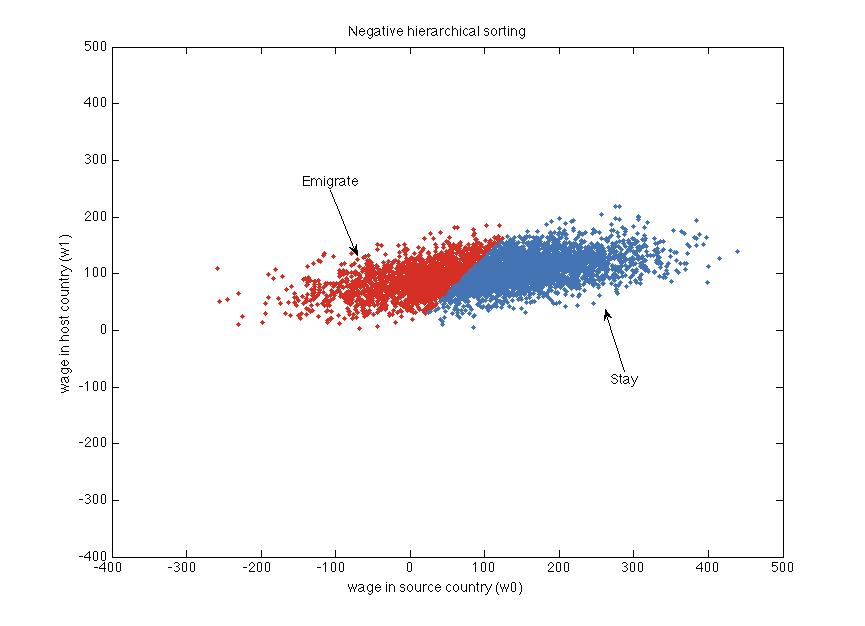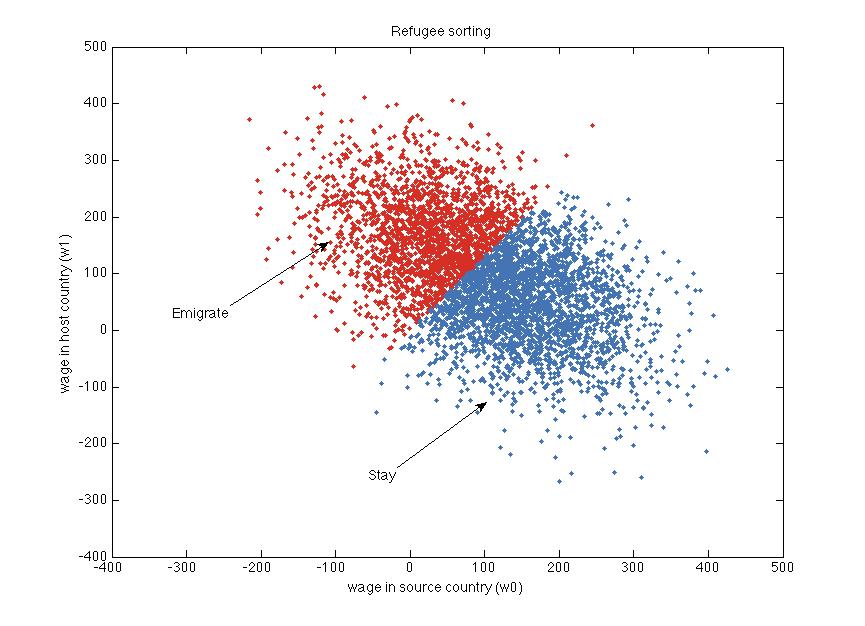# Roy model

In David Autor’s lecture notes on the Roy model he walks us through the migration choice model by Borjas (1987). In this model, agents decide between staying in the source country or migrating to a host country. The log wages in the source country ($$w_0$$) and in the host country ($$w_1$$) are given by:

$\begin{eqnarray} w_0 =& \, \mu_0 + \varepsilon_0 \\ w_1 =& \, \mu_1 + \varepsilon_1 \\ \end{eqnarray}$

The wage shocks $$\varepsilon_0$$ and $$\varepsilon_1$$ are drawn from a multivariate normal distribution and are correlated. The agents know all of these values and wages don’t adjust.

In Matlab, let’s simulate a number of agents:

We leave the two means $$\mu_0$$ and $$\mu_1$$ equal and concentrate on the effect of the relative standard deviations and the correlation. Next, we impose a cost of emigrating that rises in the source country wage and then check which agent wants to emigrate:

We can then make the following plot:Every dot is one agent. The x-axis shows their source country wages and the y-axis their host country wages. The cloud of dots is centered on (100, 100).

Agents marked red choose to emigrate and agents marked blue choose to stay. The slope of the line separating the red and blue dots is steeper, the higher cost of moving we pick.

Autor shows that there are three cases for migration. With the current settings in the simulation, we get positive hierarchical sorting. This comes about if the wage shocks are sufficiently positively correlated across countries and the wage distribution is more dispersed in the host country than in the source country. Then, only the most productive will migrate. Those who migrate have above-average wages in both the source and the host country.

We get negative hierarchical sorting, if we change sigma0 = 100 and sigma0 = 30:The wage shocks still need to be positively correlated across countries, but now the wages in the host country are more compressed than in the source country. Now, only less productive agents will migrate and emigration acts as an insurance. In this case, the mean wages (of those who choose to emigrate) is below the average of 100 in both countries.

The last case is refugee sorting, where the wage shocks are negatively correlated, so agents are below the mean income in the source country, but above the mean income in the host country. Set c = -0.5, sigma0 = 100 and sigma1 = 100 to get:Here, migrants go from below-average wages in the source country to above-average wages in the host country. This could be the case if highly productive people are suppressed in their home countries.

Autor concludes with:

The growing focus of empirical economists on applying instrumental variables to causal estimation is in large part a response to the realization that self-selection (i.e., optimizing behavior) plagues interpretation of ecological relationships. […] But instrumental variables are not the only answer to testing cause and effect with observed data. Self-selection also points to the existence of equilibrium relationships that should be observed in ecological data […], and these can be tested without an instrument. In fact, there are some natural sciences that proceed almost entirely without experimentation — for example, astrophysics. How do they do it? Models predict nonobvious relationships in data. These implications can be verified or refuted by data, and this evidence strengthens or overturns the hypotheses. Many economists seem to have forgotten this methodology.